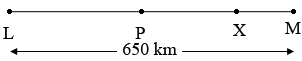SEARCH HOMEMath Central Quandaries & QueriesQuestion from abhay, a student: The speeds of John and Max are 30 km/h and 40 km/h. Initially Max is at a place L and John is at a place M. The distance between L and M is 650 km. John started his journey 3 hours earlier than Max to meet each other. If they meet each other at a place P somewhere between L and M, then the distance between P and M is?Hi,

I can help get you started. First I would draw a diagram.John starts at $M$ and walks toward Max at 30 km/h and in 3 hours is at $X.$ What is the distance from $M$ to $X?$ At this time Max starts walking at 40 km/h towards John. At what rate is the distance between them changing? How long will it take for them to meet?

I hope this helps,
PennyMath Central is supported by the University of Regina and the Imperial Oil Foundation.